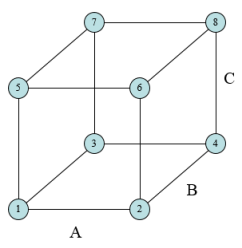Experimental design techniques are designed to discover what factors or interactions have a significant impact on a response variable.  Our SPC for Excel provides an easy-to-use design of experiments (DOE) methodology in the Excel environment you know.

The software contains two-level full factorial designs (up to 7 factors), fractional factorial designs (29 different designs, up to 15 factors), and Plackett-Burman designs (up to 27 factors).

The output includes the ANOVA table for the factors and interactions, the model in both coded and uncoded format, normal and half-normal plot of effects and much more.  A complete list of features is given below.

## Experimental Design Features

• Full factorials
• Fractional factorials
• Plackett- Burman
• Design table analysis
• ANOVA Table for factors and interactions
• ANOVA for model
• Model for coded and actual factors
• Design statistics
• Average
• Standard deviation
• Coefficient of variation
• R square
• PRESS
• R square prediction
• Normal plot of effects
• Half-normal plot of effects
• Effects charts
• Two factor charts
• Residuals analysis
• Residuals
• Leverage
• Standardized residuals
• Internally studentized residuals
• Externally studentized residuals
• DFFITS
• Cook's distance
• Potential outliers in red
• Residuals charts (raw, standardized, internally or externally studentized residuals)
• Normal plot
• Versus predicted values
• Versus actual run number
• Other charts
• Predicted vs actual
• DFFITS, Cook's distance and leverage versus actual run number
• DOE optimization chart
• Easily select effects to include/exclude in the analysis
• p values < 0.05 in red

#### SPC Knowledge BaseSign up for our FREE monthly publication featuring SPC techniques and other statistical topics.